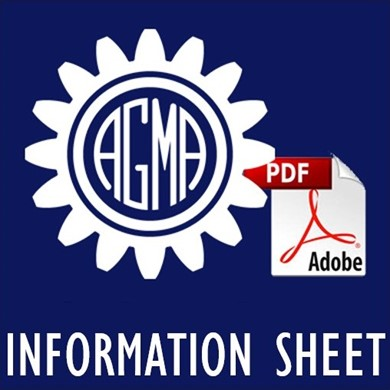CustomProperty
A Summary of Numerical Examples Demonstrating the Procedures for Calculating Geometry Factors for Spur and Helical Gears

## AGMA 918-A93SCOPE
This information sheet provides a set of numerical examples which calculate the geometry factor for pitting resistance, I, and bending strength, J, for a variety of gearsets selected to demonstrate the various gear geometries analyzed in AGMA 908-B89, Geometry Factors for Determining the Pitting Resistance and Bending Strength of Spur, Helical and Herringbone Gear Teeth. A flow chart is also included to formalize the calculation procedures for the numerical examples and to assist in the practical application of AGMA 908-B89.

Numerical Examples
Numerical examples were selected to demonstrate the following conditions: accurate and inaccurate spur gears, conventional and LACR (low axial contact ratio) helical gears, internal and external gears, double helical and herringbone (Sykes) gears and addendum modification. For simplification purposes and for demonstrating the effect on resulting geometry factors, similar examples were selected with different load locations (example 3.1.1 vs 3.1.2) and face widths (example 3.1.3 vs 3.1.4)

The results are presented in tabular form by providing the numerical results for each equation as presented in AGMA 908-B89 and appropriate to that gear geometry. Gear cutter data is presented for each component in each numerical example. All gearsets are functional and do not violate any of the exceptions stated in the scope of AGMA 908-B89. The examples used are for demonstration purposes only and are not intended to be recommendations for gearset design.

Flow Chart
The flow chart provides a step by step procedure for calculating geometry factors, I and J, using the equations and instructions from AGMA 908-B89. The numerical value tables are formatted to coincide with the flow chart procedures.

Exceptions
A procedure for the calculation of bending strength geometry factor, J, for internal gears has not been established by AGMA. For this reason, numerical examples and flow chart procedures for such a calculation are not included.

FOREWORD
[The foreword, footnotes and annexes, if any, in this document are provided for informational purposes only and are not to be construed as a part of AGMA 918-A93, A Summary of Numerical Examples Demonstrating the Procedures for Calculating Geometry Factors for Spur and Helical Gears.]

This AGMA information sheet and related publications are based on typical or average data, conditions, or application.

This information sheet, AGMA 918-A93, was prepared to assist designers in the proper use and interpretation of AGMA 908-B89 and to assist in the development of computer programs when calculating geometry factors for pitting resistance, I, and bending strength, J. A flow chart provides a step by step procedure for the calculation of these factors, either manually or by computer program. Several examples are provided to demonstrate the calculation procedure for the various characteristics of geometry as described in AGMA 908-B89.

These include accurate and inaccurate spur gears, conventional and LACR helical gears, internal and external gears, double helical and herringbone (Sykes) gears, and addendum modifications. The calculation of J-factor for internal gears is not defined in AGMA 908-B89 and, therefore, is not covered in this information sheet. A tabulation of all calculated variables is provided for each example based on its design criteria. This provides the designer with known results to check against when calculating or programming these factors.

Reaffirmed November 2020

Pages: 47

ISBN: 1-55589-617-0

Discounted member price: 65.00
130.00
You could save: 50.0%